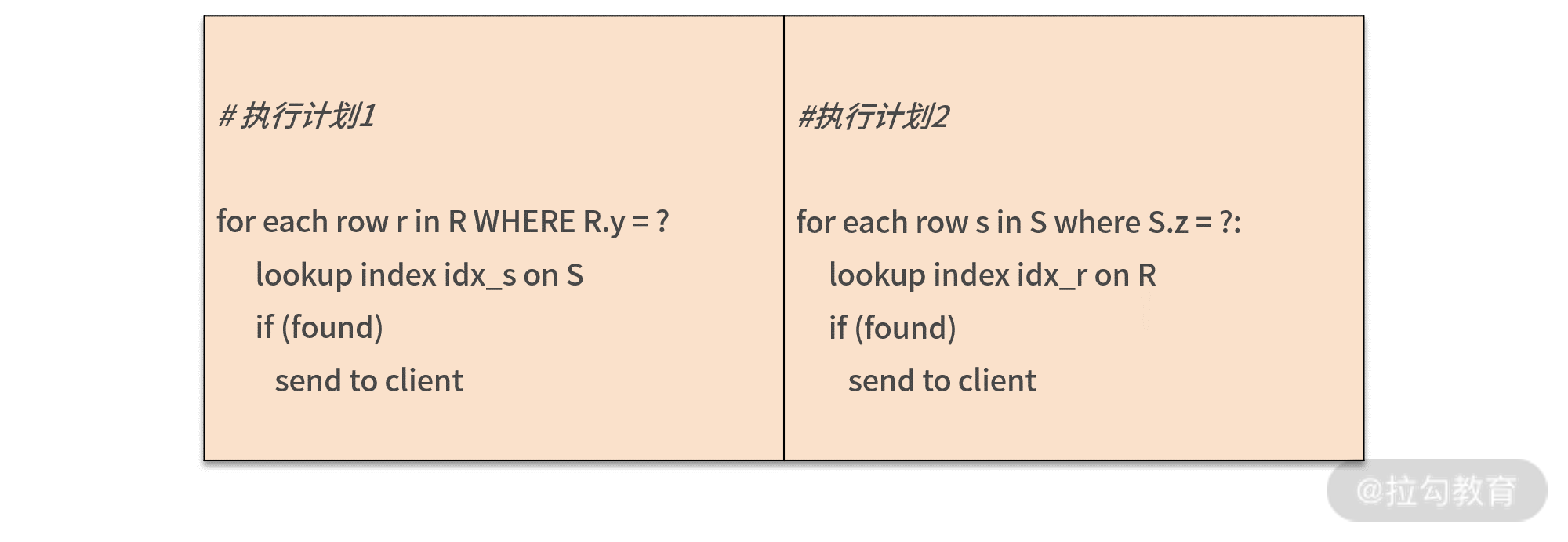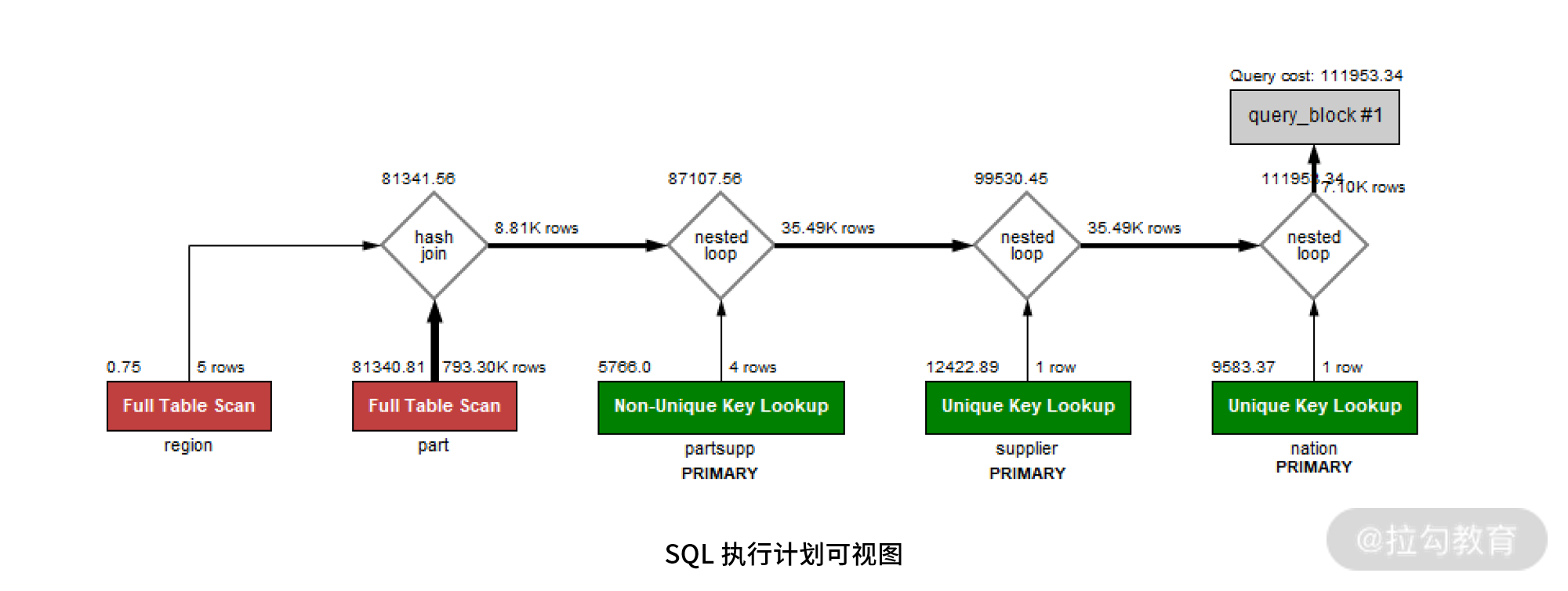12 JOIN 连接：到底能不能写 JOIN？

JOIN连接算法

MySQL 8.0 版本支持两种 JOIN 算法用于表之间的关联：

• Nested Loop Join；
• Hash Join。

Nested Loop Join

Nested Loop Join 之间的表关联是使用索引进行匹配的，假设表 R 和 S 进行连接，其算法伪代码大致如下：

for each row r in R with matching condition:

lookup index idx_s on S where index_key = r

if (found)

send to client

SELECT ... FROM R LEFT JOIN S ON R.x = S.x WEHRE ...

SELECT ... FROM R RIGHT JOIN S ON R.x = S.x WEHRE ...

SELECT ... FROM R INNER JOIN S ON R.x = S.x WEHRE ...

SELECT ... FROM R INNER JOIN S

ON R.x = S.x

WHERE R.y = ? AND S.z = ?SELECT COUNT(1)

FROM orders

INNER JOIN lineitem

ON orders.o_orderkey = lineitem.l_orderkey

WHERE orders.o_orderdate >= '1994-02-01'

AND  orders.o_orderdate < '1994-03-01'

EXPLAIN: -> Aggregate: count(1)

-> Nested loop inner join  (cost=115366.81 rows=549152)

-> Filter: ((orders.O_ORDERDATE >= DATE'1994-02-01') and (orders.O_ORDERDATE < DATE'1994-03-01'))  (cost=26837.49 rows=133612)

-> Index range scan on orders using idx_orderdate  (cost=26837.49 rows=133612)

-> Index lookup on lineitem using PRIMARY (l_orderkey=orders.o_orderkey)  (cost=0.25 rows=4)

1. Index range scan on orders using idx_orderdate：使用索引 idx_orderdata 过滤出1994 年 2 月的订单数据，预估记录数超过 13 万。
2. Index lookup on lineitem using PRIMARY：将第一步扫描的结果作为驱动表，然后将驱动表中的每行数据的 o_orderkey 值，在 lineitem 的主键索引中进行查找。
3. Nested loop inner join：进行 JOIN 连接，匹配得到的输出结果。
4. Aggregate: count(1)：统计得到最终的商品数量。

EXPLAIN FORMAT=tree

SELECT COUNT(1)

FROM orders

INNER JOIN lineitem

ON orders.o_orderkey = lineitem.l_orderkey

WHERE orders.o_orderdate >= '1994-02-01'

AND  orders.o_orderdate < '1994-03-01'

AND lineitem.l_partkey = 620758

EXPLAIN: -> Aggregate: count(1)

-> Nested loop inner join  (cost=17.37 rows=2)

-> Index lookup on lineitem using lineitem_fk2 (L_PARTKEY=620758)  (cost=4.07 rows=38)

-> Filter: ((orders.O_ORDERDATE >= DATE'1994-02-01') and (orders.O_ORDERDATE < DATE'1994-03-01'))  (cost=0.25 rows=0)

-> Single-row index lookup on orders using PRIMARY (o_orderkey=lineitem.l_orderkey)  (cost=0.25 rows=1)

Hash Join

MySQL 中的第二种 JOIN 算法是 Hash Join，用于两张表之间连接条件没有索引的情况。

1. 如果有些列是低选择度的索引，那么创建索引在导入数据时要对数据排序，影响导入性能；
2. 二级索引会有回表问题，若筛选的数据量比较大，则直接全表扫描会更快。

Hash Join算法的伪代码如下：

foreach row r in R with matching condition:

create hash table ht on r

foreach row s in S with matching condition:

search s in hash table ht:

if (found)

send to client

Hash Join会扫描关联的两张表：

• 首先会在扫描驱动表的过程中创建一张哈希表；
• 接着扫描第二张表时，会在哈希表中搜索每条关联的记录，如果找到就返回记录。

Hash Join 选择驱动表和 Nested Loop Join 算法大致一样，都是较小的表作为驱动表。如果驱动表比较大，创建的哈希表超过了内存的大小，MySQL 会自动把结果转储到磁盘。

SELECT

s_acctbal,

s_name,

n_name,

p_partkey,

p_mfgr,

s_phone,

s_comment

FROM

part,

supplier,

partsupp,

nation,

region

WHERE

p_partkey = ps_partkey

AND s_suppkey = ps_suppkey

AND p_size = 15

AND p_type LIKE '%BRASS'

AND s_nationkey = n_nationkey

AND n_regionkey = r_regionkey

AND r_name = 'EUROPE';1. 在 73390 条记录上创建基于 region 的索引，然后在内表中通过索引进行查询；
2. 对表 region 创建哈希表，73390 条记录在哈希表中进行探测；

-> Nested loop inner join  (cost=101423.45 rows=79)

-> Nested loop inner join  (cost=92510.52 rows=394)

-> Nested loop inner join  (cost=83597.60 rows=394)

-> Inner hash join (no condition)  (cost=81341.56 rows=98)

-> Filter: ((part.P_SIZE = 15) and (part.P_TYPE like '%BRASS'))  (cost=81340.81 rows=8814)

-> Table scan on part  (cost=81340.81 rows=793305)

-> Hash

-> Filter: (region.R_NAME = 'EUROPE')  (cost=0.75 rows=1)

-> Table scan on region  (cost=0.75 rows=5)

-> Index lookup on partsupp using PRIMARY (ps_partkey=part.p_partkey)  (cost=0.25 rows=4)

-> Single-row index lookup on supplier using PRIMARY (s_suppkey=partsupp.PS_SUPPKEY)  (cost=0.25 rows=1)

-> Filter: (nation.N_REGIONKEY = region.r_regionkey)  (cost=0.25 rows=0)

-> Single-row index lookup on nation using PRIMARY (n_nationkey=supplier.S_NATIONKEY)  (cost=0.25 rows=1)

OLTP 业务能不能写 JOIN？

OLTP 业务是海量并发，要求响应非常及时，在毫秒级别返回结果，如淘宝的电商业务、支付宝的支付业务、美团的外卖业务等。

SELECT o_custkey, o_orderdate, o_totalprice, p_name FROM orders,lineitem, part

WHERE o_orderkey = l_orderkey

AND l_partkey = p_partkey

AND o_custkey = ?

ORDER BY o_orderdate DESC

LIMIT 30;

SELECT * FROM orders

WHERE o_custkey = ?

ORDER BY o_orderdate DESC;

SELECT * FROM lineitem

WHERE l_orderkey = ?;

SELECT * FROM part

WHERE p_part = ?

EXPLAIN: -> Limit: 30 row(s)  (cost=27.76 rows=30)

-> Nested loop inner join  (cost=27.76 rows=44)

-> Nested loop inner join  (cost=12.45 rows=44)

-> Index lookup on orders using idx_custkey_orderdate (O_CUSTKEY=1; iterate backwards)  (cost=3.85 rows=11)

-> Index lookup on lineitem using PRIMARY (l_orderkey=orders.o_orderkey)  (cost=0.42 rows=4)

-> Single-row index lookup on part using PRIMARY (p_partkey=lineitem.L_PARTKEY)  (cost=0.25 rows=1)

总结

MySQL 数据库中支持 JOIN 连接的算法有 Nested Loop Join 和 Hash Join 两种，前者通常用于 OLTP 业务，后者用于 OLAP 业务。在 OLTP 可以写 JOIN，优化器会自动选择最优的执行计划。但若使用 JOIN，要确保 SQL 的执行计划使用了正确的索引以及索引覆盖，因此索引设计显得尤为重要，这也是DBA在架构设计方面的重要工作之一。